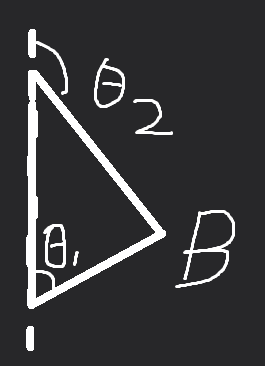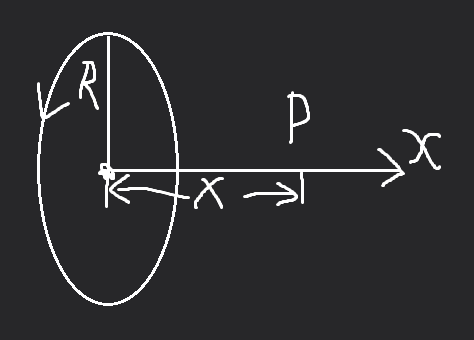# 大学物理

• 在球内部 ( $r < R$ ) ，场强$E=0$，电势$U$与球面相同（与$r$无关）
• 空腔内无电荷，电荷分布在外表面上（内表面无电荷）
• 球壳实部场强$E=0$
• 磁力矩$P_m$与磁场$B$方向一致时稳定

## 第11章​

• 毕奥萨法尔定律：$d\vec{B}=\dfrac{μ_0}{4π}\dfrac{Id\vec{l}\times\vec{e}}{r^2}$ 不均匀磁场：$\vec{B}=\int d\vec{B}$
• 运动电荷的磁场：$d\vec{B}=\dfrac{μ_0}{4π}\dfrac{q\vec{v}\times\vec{e}}{r^2}$
• 磁场的高斯定理：$\oint_S\vec{B}·d\vec{S}=0$
• 安培环路定理：$\oint_l\vec{B}·d\vec{l}=μ_0\sum I$（a：$θ_1$顶点到$θ_2$顶点的距离）

$θ_1$：起始端与电流方向夹角）

$θ_2$：末端与电流方向夹角）